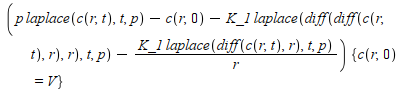## 45 Reputation

1 years, 240 days

## How to automate discretization of high o...

Maple

Hello,

I am trying to discretize high order derivatives (typicaly of order 3-4) and I would like to use maple to avoid errors. Using this thread of discussion I got the following minimum example that illustrates the problem I am facing:> # central discretisation u_xx, i=1,..,n-1 dxc:=add([1,-2,1] *~ [u[i+k,j] \$k=-1..1])/(h^2);(1)> # we need to compute gradient(laplacian) on faces i+1/2,j and i-1/2,j.
 > #First diff(u_xx,x)|i+1/2,j d1:=add([1,-1] *~ [dxc[i+p,j] \$p=0..1])/(h); #which should give d1:=add([1,3,-3,1] *~ [u[i+k,j] \$k=-1..2])/(h^3);(3)I would like maple to evaluate the first expression for d1 and give the second expression that I wrote manually.

Is there a way to do this ? (using simplify() does't produce anything)

Cheers,

## Cannot solve with dsolve the self simila...

Maple 2021

Hello,

I am trying to solve the self similarity problem for external natural convection which is the following coupled problem

•     3/4*F(eta)*Theta(eta)'  = Theta(eta)''
•     1/2 (F(eta)')^2 - 3/4 F(eta)*F(eta)'' = Pr*(Theta(eta) - F(eta)''')

where Pr is a constant and  ()' denotes differentiation with respect to eta. The boundary conditions are:

•     at eta = 0, F = 0, F'=0, Theta = 1
•     at eta -> infinity, F' = 0, Theta = 0

I tried the following but I cannot obtain a solution> Pr:=1(1)
 > etamax := infinity(2)> eq:= 3/4*F(eta)*diff(theta(eta),eta) = diff(theta(eta),eta,eta),1/Pr*(1/2*diff(F(eta),eta)^2 - 3/4*F(eta)*diff(F(eta),eta,eta)) = -diff(F(eta),eta,eta,eta) + theta(eta)(3)>> bcs := F(0) = 0, theta(0)=1, D(F)(0)=0,theta(etamax)=0,D(F)(etamax)=0(4)> sol:=dsolve([eq,bcs],[F(eta),theta(eta)])## eval is not evaluating expression, is th...

Maple
Hello Iam following this tutorial to solve pde with laplace transforms  but have a problem with eval which is not evaluating the following expression
```restart;
with(PDEtools);
with(inttrans);

# Equations for c in physical space
PDE1 := diff(c(r, t), t) = K_1*diff(r*diff(c(r, t), r), r)/r;

#initial condition
ic1 := c(r, 0) = V

# Equation in Laplace space
PDEL1 := laplace((lhs - rhs)(PDE1), t, p);

# Apply initial condition to simplify
simplified_PDEL1 := eval(PDEL1*{ic1})```

which is displayed (an treated) aswith c(r,0) not being replaced by V. When I try to solve the problem with dsolve I get the following error

`Error, (in dsolve) invalid input: `PDEtools/NumerDenom` expects its 1st argument, ee, to be of type algebraic, but received {c(r, 0) = V}`

If I replace manually C(r,0) by V then the error dissapears. Is this how eval is supposed to behave ?

Cheers,

## Can't solve the linear pde problem of "t...

Maple

Hello,

I am trying to recover with maple the analytical solution of the problem of conduction within two composite cylinders given in Carslaw and Jeager's book: "Conduction of heat in solids" (link here). Here is my take on the problem

```restart;
with(PDEtools);
C := diff_table(c(rho, t));
E := diff_table(e(rho, t));
PDE1 := diff(C[], t) - K_1*(diff(diff(C[], rho), rho) - diff(C[], rho)/rho) = 0;
PDE2 := diff(E[], t) - K_2*(diff(diff(E[], rho), rho) - diff(E[], rho)/rho) = 0;

ic1 := eval(C[], t = 0) = V;
ic2 := eval(E[], t = 0) = 0;

bc_1 := eval(C[], rho = a) = eval(E[], rho = a);
bc_2 := K_1*eval(diff(C[], rho), rho = a) = K_2*eval(diff(E[], rho), rho = a);
bc_3 := eval(E[], rho = infinity) = 0;
bc_4 := (diff(C[]/rho, rho), rho = 0) = 0;
pdsolve([PDE1, PDE2],[ic1,ic2, bc_1,bc_2,bc_3,bc_4]); ```

Unfortunately I end up with the following errors:

```Error, (in pdsolve/sys) too many arguments; some or all of the following are wrong: [{c(rho, t), e(rho, t)}, [c(rho, 0) = V, e(rho, 0) = 0, c(a, t) = e(a, t), K_1*(diff(c(a, t), a)) = K_2*(diff(e(a, t), a)), e(infinity, t) = 0, ((diff(c(rho, t), rho))/rho-c(rho, t)/rho^2, rho = 0) = 0]]
```

If I call pdsolve without any initial and boundary conditions, I have the general form of the equation which is correct (product of exponential function in time with Bessel functions for space) but I don't know how to determine the coeficients with maple from there.

Note also that the boundary condition "bc_2" does not seem to be correcty evaluated (it is supposed to specify the continuity of the flux ar r=a). Instead it just evaluate the expression with r swapped with a. If one write the same line of code with r=0 instead of r=a then the evaluation seems correct.

Any help would be very appreciated,

Cheers

## solve ode where unknowns have a dependen...

Maple 2019

Hello,

I am trying to solve the following system of odes where the unknowns are xhat, yhat, uhat and vhat. They depend on the variables (x, y, u, v, a_1, a_2, a_3, a_4, f(x)) where f(x) is an arbitrary function and I want to solve it with respect to a_1. The RHS has f(x) and its derivative with respect to x. The dsolve function fails to solve the ode when I add f(x) at the list of variables.

```restart;
vars := x, y, u, v, a_1, a_2, a_3, a_4, f(x);
sys_ode := diff(xhat(vars), a_1) = a_3*x + a_1, diff(yhat(vars), a_1) = a_2 - a_4*y + f(x), diff(uhat(vars), a_1) = a_3*u + 2*a_4*u, diff(vhat(vars), a_1) = a_4*v + diff(f(x), x)*u;

initvars := x, y, u, v, 0, a_2, a_3, a_4, f(x);
ics := xhat(initvars) = x, yhat(initvars) = y, uhat(initvars) = u, vhat(initvars) = v;
solution_a1 = dsolve([sys_ode, ics], [xhat, yhat, uhat, vhat]);```

Can anyone help regarding this issue ?

 Page 1 of 1
﻿